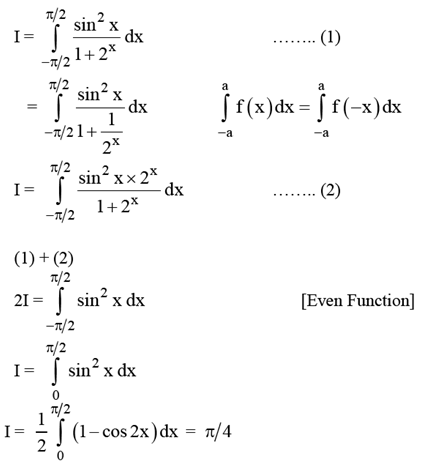Checkout JEE MAINS 2022 Question Paper Analysis : Checkout JEE MAINS 2022 Question Paper Analysis :

# The value of $$\int_{\frac{-\pi }{2}}^{\frac{\pi }{2}}\frac{\sin ^{2}x}{1+2^{x}}dx$$ is:

a) 4π

b) π/4

c) π/8

d) π/2

Solution:(0)(0)